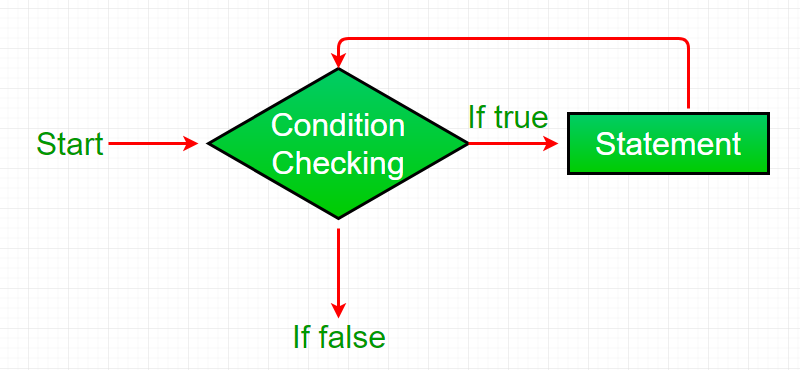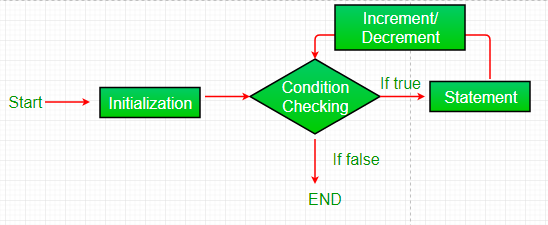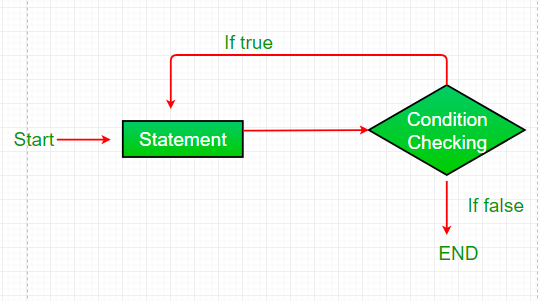GFG App
Open AppBrowser
Continue

# Loops in Java

Looping in programming languages is a feature which facilitates the execution of a set of instructions/functions repeatedly while some condition evaluates to true. Java provides three ways for executing the loops. While all the ways provide similar basic functionality, they differ in their syntax and condition checking time.

java provides Three types of Conditional statements this second  type is loop statement .

• while loop: A while loop is a control flow statement that allows code to be executed repeatedly based on a given Boolean condition. The while loop can be thought of as a repeating if statement.

Syntax :

```while (boolean condition)
{
loop statements...
}```

## Java

 `/*package whatever //do not write package name here */`   `import` `java.io.*;`   `class` `GFG {` `    ``public` `static` `void` `main (String[] args) {` `      ``int` `i=``0``;` `      ``while` `(i<=``10``)` `      ``{` `        ``System.out.println(i);` `        ``i++;` `      ``}` `    ``}` `}`

Output

```0
1
2
3
4
5
6
7
8
9
10```
• Flowchart:• While loop starts with the checking of Boolean condition. If it evaluated to true, then the loop body statements are executed otherwise first statement following the loop is executed. For this reason it is also called Entry control loop
• Once the condition is evaluated to true, the statements in the loop body are executed. Normally the statements contain an update value for the variable being processed for the next iteration.
• When the condition becomes false, the loop terminates which marks the end of its life cycle.
• for loop: for loop provides a concise way of writing the loop structure. Unlike a while loop, a for statement consumes the initialization, condition and increment/decrement in one line thereby providing a shorter, easy to debug structure of looping.

Syntax:

```for (initialization condition; testing condition;increment/decrement)
{
statement(s)
}```

## Java

 `/*package whatever //do not write package name here */`   `import` `java.io.*;`   `class` `GFG {` `    ``public` `static` `void` `main (String[] args) {` `       ``for` `(``int` `i=``0``;i<=``10``;i++)` `       ``{` `         ``System.out.println(i);` `       ``}` `    ``}` `}`

Output

```0
1
2
3
4
5
6
7
8
9
10```
• Flowchart:• Initialization condition: Here, we initialize the variable in use. It marks the start of a for loop. An already declared variable can be used or a variable can be declared, local to loop only.
• Testing Condition: It is used for testing the exit condition for a loop. It must return a boolean value. It is also an Entry Control Loop as the condition is checked prior to the execution of the loop statements.
• Statement execution: Once the condition is evaluated to true, the statements in the loop body are executed.
• Increment/ Decrement: It is used for updating the variable for next iteration.
• Loop termination:When the condition becomes false, the loop terminates marking the end of its life cycle.
• do while: do while loop is similar to while loop with only difference that it checks for condition after executing the statements, and therefore is an example of Exit Control Loop.

Syntax:

```do
{
statements..
}
while (condition);```

## Java

 `/*package whatever //do not write package name here */`   `import` `java.io.*;`   `class` `GFG {` `    ``public` `static` `void` `main (String[] args) {` `      ``int` `i=``0``;` `      ``do` `      ``{` `        ``System.out.println(i);` `        ``i++;` `      ``}``while``(i<=``10``);` `    ``}` `}`

Output

```0
1
2
3
4
5
6
7
8
9
10```
• Flowchart:• do while loop starts with the execution of the statement(s). There is no checking of any condition for the first time.
• After the execution of the statements, and update of the variable value, the condition is checked for true or false value. If it is evaluated to true, next iteration of loop starts.
• When the condition becomes false, the loop terminates which marks the end of its life cycle.
• It is important to note that the do-while loop will execute its statements atleast once before any condition is checked, and therefore is an example of exit control loop.

Pitfalls of Loops

• Infinite loop: One of the most common mistakes while implementing any sort of looping is that it may not ever exit, that is the loop runs for infinite time. This happens when the condition fails for some reason. Examples:
• Infinite for loop :

## Java

 `/*package whatever //do not write package name here */`   `import` `java.io.*;`   `class` `GFG {` `    ``public` `static` `void` `main(String[] args)` `    ``{` `        ``for` `(;;) {` `        ``}` `    ``}` `}`

infinite while loop:

## Java

 `/*package whatever //do not write package name here */`   `import` `java.io.*;`   `class` `GFG {` `    ``public` `static` `void` `main (String[] args) {` `       ``while` `(``true``)` `       ``{` `         ``// statement ` `       ``}` `      `  `    ``}` `}`

## Java

 `//Java program to illustrate various pitfalls.` `public` `class` `LooppitfallsDemo` `{` `    ``public` `static` `void` `main(String[] args)` `    ``{`   `        ``// infinite loop because condition is not apt` `        ``// condition should have been i>0.` `        ``for` `(``int` `i = ``5``; i != ``0``; i -= ``2``)` `        ``{` `            ``System.out.println(i);` `        ``}` `        ``int` `x = ``5``;`   `        ``// infinite loop because update statement` `        ``// is not provided.` `        ``while` `(x == ``5``)` `        ``{` `            ``System.out.println("In the loop");` `        ``}` `    ``}` `}`

Another pitfall is that you might be adding something into you collection object through loop and you can run out of memory. If you try and execute the below program, after some time, out of memory exception will be thrown.

## Java

 `//Java program for out of memory exception.` `import` `java.util.ArrayList;` `public` `class` `Integer1` `{` `    ``public` `static` `void` `main(String[] args)` `    ``{` `        ``ArrayList ar = ``new` `ArrayList<>();` `        ``for` `(``int` `i = ``0``; i < Integer.MAX_VALUE; i++)` `        ``{` `            ``ar.add(i);` `        ``}` `    ``}` `}`

Output:

```Exception in thread "main" java.lang.OutOfMemoryError: Java heap space
at java.util.Arrays.copyOf(Unknown Source)
at java.util.Arrays.copyOf(Unknown Source)
at java.util.ArrayList.grow(Unknown Source)
at java.util.ArrayList.ensureCapacityInternal(Unknown Source)
at article.Integer1.main(Integer1.java:9)```

Nested Loop:

Nested loop means a loop statement inside another loop statement.

There are different combinations of loop using for loop, while loop, do-while loop.

Ex.1 Nested for loop

## Java

 `/*package whatever //do not write package name here */`   `import` `java.io.*;`   `class` `GFG {` `    ``public` `static` `void` `main (String[] args) {` `        ``for``(``int` `i = ``0``; i < ``3``; i++){` `          ``for``(``int` `j = ``0``; j < ``2``; j++){` `            ``System.out.println(i);` `          ``}` `          ``System.out.println();` `        ``}` `    ``}` `}`

Output

```0
0

1
1

2
2```

Ex.2 Nested while loop

## Java

 `import` `java.io.*;`   `class` `GFG {` `    ``public` `static` `void` `main(String[] args)` `    ``{` `        ``int` `i = ``1``, j = ``1``;` `        ``while` `(i <= ``3``) {` `            ``while` `(j <= ``3``) {` `                ``System.out.print(j);` `                ``j++;` `            ``}` `            ``i++;` `            ``System.out.println(``""``);` `            ``j = ``1``;` `        ``}` `    ``}` `}`

Output

```123
123
123```

Ex.3 Nested do while loop

## Java

 `/*package whatever //do not write package name here */`   `import` `java.io.*;`   `class` `GFG {` `    ``public` `static` `void` `main(String[] args)` `    ``{`   `        ``int` `row = ``1``, column = ``1``;` `        ``int` `x;` `        ``do` `{` `            ``x = ``4``;` `            ``do` `{` `                ``System.out.print(``""``);` `                ``x--;` `            ``} ``while` `(x >= row);` `            ``column = ``1``;` `            ``do` `{` `                ``System.out.print(column + ``" "``);` `                ``column++;`   `            ``} ``while` `(column <= ``5``);` `            ``System.out.println(``" "``);` `            ``row++;` `        ``} ``while` `(row <= ``5``);` `    ``}` `}`

Output

```1 2 3 4 5
1 2 3 4 5
1 2 3 4 5
1 2 3 4 5
1 2 3 4 5  ```

Ex.4 Nested while and for loop

## Java

 `/*package whatever //do not write package name here */`   `import` `java.io.*;`   `class` `GFG {` `    ``public` `static` `void` `main(String[] args)` `    ``{`   `        ``int` `weeks = ``3``;` `        ``int` `days = ``7``;` `        ``int` `i = ``1``;`   `        ``// outer loop` `        ``while` `(i <= weeks) {` `            ``System.out.println(``"Week: "` `+ i);`   `            ``// inner loop` `            ``for` `(``int` `j = ``1``; j <= days; ++j) {` `                ``System.out.println(``"  Days: "` `+ j);` `            ``}` `            ``++i;` `        ``}` `    ``}` `}`

Output

```Week: 1
Days: 1
Days: 2
Days: 3
Days: 4
Days: 5
Days: 6
Days: 7
Week: 2
Days: 1
Days: 2
Days: 3
Days: 4
Days: 5
Days: 6
Days: 7
Week: 3
Days: 1
Days: 2
Days: 3
Days: 4
Days: 5
Days: 6
Days: 7```